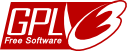# The Octonion RPN Calculator

###Software © (2009) John Wayland Bales under the GNU General Public License

You may use or modify this software for any non-commercial use so long as you include this copyright notice.

Also check out the Sedenion calculator.

The calculator may be operated in the context of five different algebras, the traditional eight-square octonion algebra based on Degan's eight square identity*, or one of four isomorphic algebras based on Cayley-Dickson doubling formulas.

This is a 'Reverse Polish octonion calculator' with a level three pushdown stack. The top of the stack is the rightmost column. The traditional 'PUSH' operation is here rendered as 'ENTER'

Octonions may be entered by hand in the top register or you may generate random entries and store them for reuse.

To perform an operation such as x + y you would do x enter y plus and the result would appear in the top register.

An example of a more complex calculation is given below the calculator.

There are sixteen memory registers X,Y,Z plus A through M accessible from a dropdown list.

 Degan's 8-square id P0: (ac - d*b, da + bc*) P1: (ac - db*, cb + a*d ) P2: (ca - b*d, bc + da* ) P3: (ca - bd*, ad + c*b)
Octonion Calculator
 Register 2 Register 1 Top Register e0 e1 e2 e3 e4 e5 e6 e7
 X Y Z A B C D E F G H I J K L M X Y Z A B C D E F G H I J K L M

Special note: There are different ways of numbering the unit basis vectors.

In terms of ordered pairs for the four doubling products, these basis vectors are defined recursively by

1. e0=1
2. e2k=(ek,0)
3. e2k+1=(0,ek)

#### A worked example of use

To illustrate one of the Moufang identities using randomly generated entries, for example Z(X(ZY)) = ((ZX)Z)Y, perform the following operations:

1. Select random vectors X, Y and Z
1. INPUT X STO
2. INPUT Y STO
3. INPUT Z STO
2. Perform the operations on the left side of the identity
1. Z RCL ENTER (Since Z should already be in the register, you don't actually have to recall it.)
2. Y RCL × ENTER
3. X RCL SWAP × ENTER
4. Z RCL SWAP ×
3. Store the result
1. A STO
4. Perform the operations on the right side of the identity
1. Z RCL ENTER
2. X RCL × ENTER
3. Z RCL × ENTER
4. Y RCL × ENTER
5. Subtract the result obtained on the left side of the identity. Result should be 0 (allowing for tiny round off errors of order 10-14).
1. A RCL –

*The permutation (2 3)(4 7 5 6) applied to the subscripts of the Degan product basis vectors gives the subscripts of the corresponding P3 basis vectors.

#### Here is another exercise you might try.

Notice that the formula for product P2 appears to be just the product P0 applied to (c,d)(a,b).

Likewise, for P3 and P1. So one might be excused for expecting P0:XY=P2:YX and P1:XY=P3:YX.

However, this is not the case.

In fact, P0:XY=P1:YX and P2:XY=P3:YX for octonions (but not for sedenions).

Fano Plane diagram corresponding to this calculator

Contact:John Wayland Bales, Department of Mathematics (Retired), Tuskegee University, Tuskegee, AL 36088 USA